Test: Transfer Function - 1

# Test: Transfer Function - 1

Test Description

## 20 Questions MCQ Test GATE Electrical Engineering (EE) 2023 Mock Test Series | Test: Transfer Function - 1

Test: Transfer Function - 1 for Electronics and Communication Engineering (ECE) 2023 is part of GATE Electrical Engineering (EE) 2023 Mock Test Series preparation. The Test: Transfer Function - 1 questions and answers have been prepared according to the Electronics and Communication Engineering (ECE) exam syllabus.The Test: Transfer Function - 1 MCQs are made for Electronics and Communication Engineering (ECE) 2023 Exam. Find important definitions, questions, notes, meanings, examples, exercises, MCQs and online tests for Test: Transfer Function - 1 below.
Solutions of Test: Transfer Function - 1 questions in English are available as part of our GATE Electrical Engineering (EE) 2023 Mock Test Series for Electronics and Communication Engineering (ECE) & Test: Transfer Function - 1 solutions in Hindi for GATE Electrical Engineering (EE) 2023 Mock Test Series course. Download more important topics, notes, lectures and mock test series for Electronics and Communication Engineering (ECE) Exam by signing up for free. Attempt Test: Transfer Function - 1 | 20 questions in 60 minutes | Mock test for Electronics and Communication Engineering (ECE) preparation | Free important questions MCQ to study GATE Electrical Engineering (EE) 2023 Mock Test Series for Electronics and Communication Engineering (ECE) Exam | Download free PDF with solutions
 1 Crore+ students have signed up on EduRev. Have you?
Test: Transfer Function - 1 - Question 1

### The transfer function of given system is: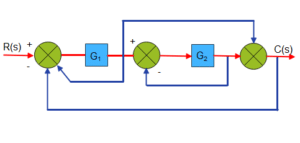Detailed Solution for Test: Transfer Function - 1 - Question 1

The signal flow graph given below.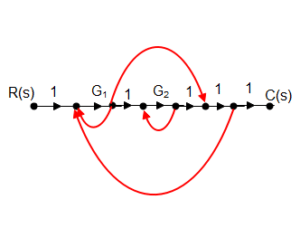The transfer function=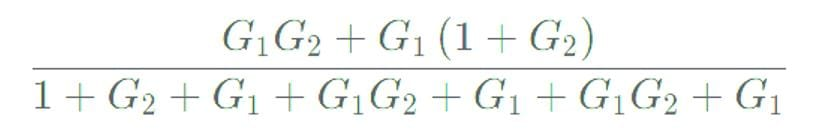=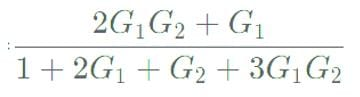Test: Transfer Function - 1 - Question 2

### The output of the feedback control system must be a function of:

Detailed Solution for Test: Transfer Function - 1 - Question 2

Explanation: In a feedback control system, the output is determined by a combination of the input signal and the feedback signal. The input signal is the desired output or reference, while the feedback signal is a portion of the actual output that is fed back into the system to compare with the input. This comparison helps the system to adjust its output to minimize the error between the input and the actual output. By considering both the input and feedback signals, the system can continuously adapt and achieve the desired output.

Test: Transfer Function - 1 - Question 3

### The unit impulse response of a certain system is found to be e-8t. Its transfer function is _______.

Detailed Solution for Test: Transfer Function - 1 - Question 3

The impulse response is defined as the output of an LTI system due to a unit impulse signal input being applied at time t = 0.

y(t) = h(t) x(t) = h(t) δ(t)

where δ(t) is the unit impulse function and h(t) is the unit impulse response of a continuous-time LTI system.

Calculations:-

Given-

y(t)  = e-8t

x(t) = δ(t)

For calculating the transfer function convert the time domain response into Laplace or S domain.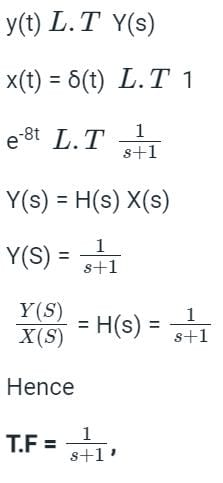*Answer can only contain numeric values
Test: Transfer Function - 1 - Question 4

For the system shown in the figure, Y(s)/X(s) = _________. (Answer in integer )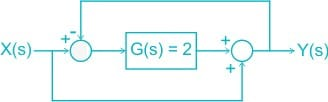Detailed Solution for Test: Transfer Function - 1 - Question 4

The circuit is redrawn as shown: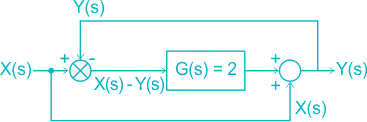[X(s) – Y(s)] G(s) + X(s) = Y(s)

Given G(s) = 2

(X(s) – Y(s)) 2 + X(s) = Y(s)

= 2 X(s) + X(s) = Y(s) + 2Y(s)

= 3 X(s) = 3 Y(s)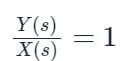Test: Transfer Function - 1 - Question 5

Assuming zero initial condition, the response y(t) of the system given below to a unit step input u(t) is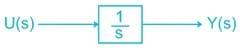Detailed Solution for Test: Transfer Function - 1 - Question 5

Laplace transform of u(t) is given by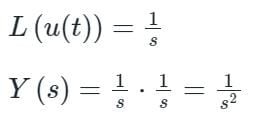Taking Inverse Laplace transform

y(t) = t.u(t)

Test: Transfer Function - 1 - Question 6

The sum of the gains of the feedback paths in the signal flow graph shown in fig.  is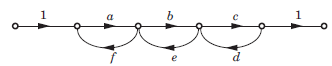Detailed Solution for Test: Transfer Function - 1 - Question 6
• Any feedback path is considered as a valid feedback path if it does not touches any single node twice in its path.
• Feedback paths in the above diagram are af, be, cd, abef, bcde, and abcdef.
• abef is not a valid feedback path because node 'Q' is repeated twice in the path.
• bcde is not a valid feedback path because node 'R' is repeated twice in the path.
• abcdef is not a valid feedback path because node 'Q' and 'R' is repeated twice in the path.So, af, be and cd are the only valid feedback paths. The sum of the gains of the feedback paths in the signal flow graph is af + be + cd.

Test: Transfer Function - 1 - Question 7

A control system whose step response is -0.5(1+e-2t) is cascaded to another control block whose impulse response is e-t. What is the transfer function of the cascaded combination?

Detailed Solution for Test: Transfer Function - 1 - Question 7

Let's first find the transfer function of the first control system. We know that the step response is given by: y(t) = -0.5(1+e^(-2t))

Taking the Laplace transform of both sides, we get: Y(s) = -0.5(1/s + 1/(s+2))

The transfer function of the first control system is therefore: G1(s) = Y(s)/U(s) = -0.5/(s(s+2))

Now let's find the transfer function of the second control block. We know that the impulse response is given by: h(t) = e^(-t)

Taking the Laplace transform of both sides, we get: H(s) = 1/(s+1)

The transfer function of the second control system is therefore: G2(s) = H(s)/U(s) = 1/(s+1)

The transfer function of the cascaded combination is given by the product of the individual transfer functions: G(s) = G1(s) * G2(s) = [-0.5/(s(s+2))] * [1/(s+1)] = -0.5/(s(s+2)(s+1))

Therefore, the correct answer is:  1/(s+2)(s+1).

Test: Transfer Function - 1 - Question 8

The overall transfer function C/R of the system shown in fig. will be: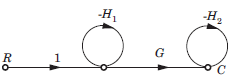Detailed Solution for Test: Transfer Function - 1 - Question 8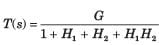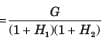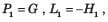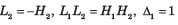Test: Transfer Function - 1 - Question 9

Consider the List I and List II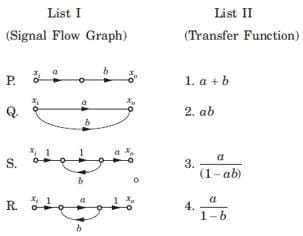The correct match is

Detailed Solution for Test: Transfer Function - 1 - Question 9
• P. P1 = ab, Δ = 1, L = 0 ,T = ab
• Q. P1 = a, P2 = 6 , Δ = 1, L = Δk = 0,T  = a+b
• R. P1 = a, L1 = b, Δ = 1 - b, Δ1 =1,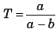• S. P1 = a, L1 = ab, Δ = 1 - ab, Δ1 = 1,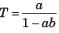Test: Transfer Function - 1 - Question 10

For the signal flow graph shown in fig. an equivalent graph is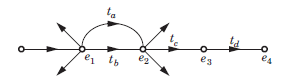Detailed Solution for Test: Transfer Function - 1 - Question 10

While writing the transfer function of this signal flow graph,

e2= tae1 + tbe= (ta+ tb) e1

Then, signal flow graph will lokk like this: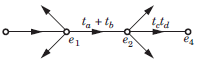Test: Transfer Function - 1 - Question 11

The block diagram of a system is shown in fig. The closed loop transfer function of this system is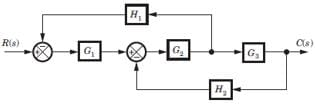Detailed Solution for Test: Transfer Function - 1 - Question 11

Consider the block diagram as SFG. There are two feedback loop -G1G2H1 and -G2G3H2 and one forward path G1G2 G3 . So (D) is correct option.

Test: Transfer Function - 1 - Question 12

For the system shown in fig. transfer function C(s) R(s) is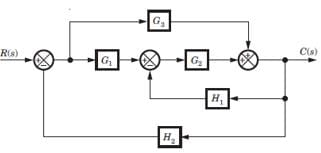Detailed Solution for Test: Transfer Function - 1 - Question 12

Consider the block diagram as a SFG. Two forward path G1G2 and G3 and three loops -G1G2 H2, -G2H1, -G3 H2
There are no nontouching loop. So (B) is correct.

Test: Transfer Function - 1 - Question 13

In the signal flow graph shown in fig. the transfer function is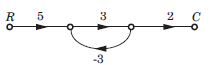Detailed Solution for Test: Transfer Function - 1 - Question 13

P1 = 5 x 3 x 2 = 30, Δ = 1 - (3x - 3) = 10
Δ1 = 1,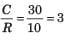Test: Transfer Function - 1 - Question 14

In the signal flow graph shown in fig. the gain C/R is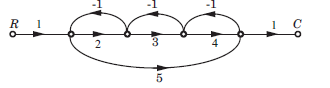Detailed Solution for Test: Transfer Function - 1 - Question 14

P1 = 2 x 3 x 4 = 24 , P2 = 1 x 5 x 1 = 5
L1 = -2, L2 = -3, L3 = -4, L4 = -5,
L1L3 = 8, Δ = 1 -(-2 - 3 - 4 - 5) + 8 = 23, Δ1 = 1, Δ2 = 1 - (-3) = 4,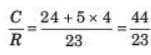Test: Transfer Function - 1 - Question 15

The gain C(s)/R(s) of the signal flow graph shown in fig.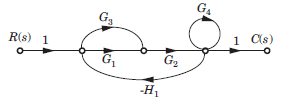Detailed Solution for Test: Transfer Function - 1 - Question 15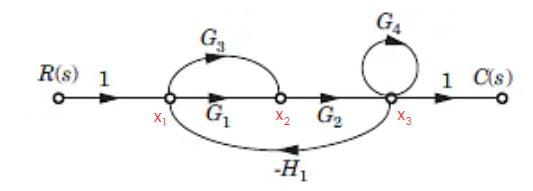X1 = 1 - H1

X2 = (G1 + G3) X1 - X3H1

X= X2G2 + G4

Test: Transfer Function - 1 - Question 16

The negative feedback closed-loop system was subjected to 15V. The system has a forward gain of 2 and a feedback gain of 0.5. Determine the output voltage and the error voltage.

Detailed Solution for Test: Transfer Function - 1 - Question 16

Given:
G(s) = 2
H(s) = 0.5 and R(s) = 10V
Output voltage: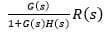= (2/1 + 2 x 0.5) x 15 = 15V
Error voltage: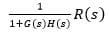= (1/1 + 2 x 0.5) x 15 = 7.5V

Test: Transfer Function - 1 - Question 17

For the block diagram shown in fig. transfer function C(s)/R(s) is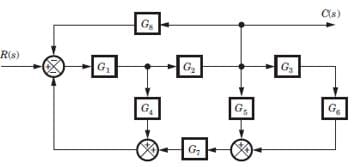Detailed Solution for Test: Transfer Function - 1 - Question 17

Four loops -G1G4, -G1G2G5, -G1,G2G5G7 and -G1G2G3G3G7.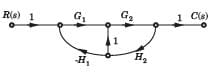There is no nontouching loop. So (B) is correct.

Test: Transfer Function - 1 - Question 18

For the block diagram shown in fig. the numerator of transfer function is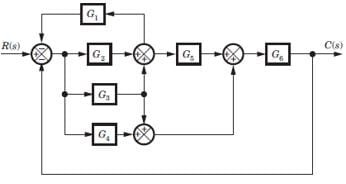Detailed Solution for Test: Transfer Function - 1 - Question 18

SFG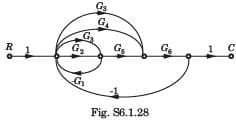P1 = G2G5G6 , P2 = G3G5G6, P3 = G3G6 , P4 = G4G6
If any path is deleted, there would not be any loop.
Hence Δ1 = Δ2 = Δ3 = Δ4 = 1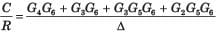Test: Transfer Function - 1 - Question 19

For the block diagram shown in fig.  the transfer function C(s)/R(s) is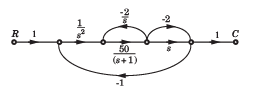Detailed Solution for Test: Transfer Function - 1 - Question 19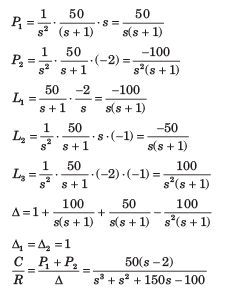Test: Transfer Function - 1 - Question 20

In the signal flow graph of figure y/x equals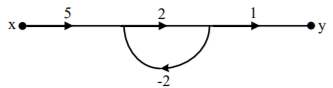Detailed Solution for Test: Transfer Function - 1 - Question 20

Transfer function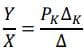PK = 5 x 2 x 1 = 10
ΔK = 1
Δ = 1 - (-4) = 5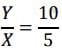= 2

## GATE Electrical Engineering (EE) 2023 Mock Test Series

22 docs|274 tests
Information about Test: Transfer Function - 1 Page
In this test you can find the Exam questions for Test: Transfer Function - 1 solved & explained in the simplest way possible. Besides giving Questions and answers for Test: Transfer Function - 1, EduRev gives you an ample number of Online tests for practice

## GATE Electrical Engineering (EE) 2023 Mock Test Series

22 docs|274 tests(Scan QR code)